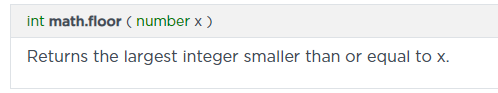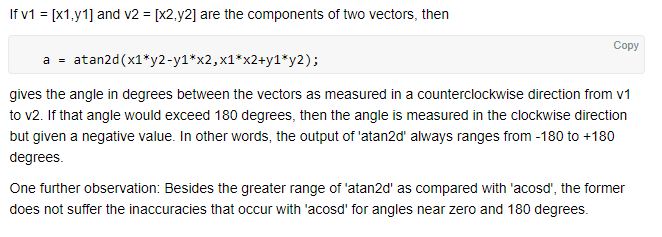# Hello World!

I am attempting to check the difference in rotation (in degrees) of two CFrames in a clockwise rotation.

I have been unable to find any resources on this specific issue, although I have found many topics that were VERY similar. I searched Scripting Helpers, here, google, and tried some of my own fruitless experiments. I even tried all 3 solutions in this post. So far I have this:

``````local Facing = Building.PrimaryPart.CFrame.LookVector
local Vector = OldBuilding.PrimaryPart.CFrame.LookVector
local Angle = math.acos(Facing:Dot(Vector))
local Degrees = math.deg(Angle)
``````

This returns the amount of degrees of difference, but the issue is that it will only report it in the nearest direction, not clockwise (e.g. Both 90 and 270 return as 90 degree difference). I want to get the difference in degrees in a clockwise motion (e.g. 90, 180, 270, 360).

Edit: It would also suffice if the counterclockwise 90 degrees would report as -90. Either solution would be welcome!

Am I going to be able to utilize what I have here, or am I not even close? I have a hard time understanding math with CFrames. I feel like what I have here may only need a tiny adjustment. Any assistance is appreciated!

PS: If anyone knows a better way to round the degrees to the nearest 90, please advise!

``````local RoundOptions = {0, 90, 180, 270, 360}
local ClosestOption = 0
for i, roundOption in ipairs(RoundOptions) do
if math.abs(Degrees - roundOption) < math.abs(Degrees - ClosestOption) then
ClosestOption = roundOption
end
end
``````

I need it to return to the nearest 90, precisely. Without this it was returning values very close, but not precise. My concern is that over enough rotations my Building would no longer be lined up with the Grid I have.

1 Like

I’m not very good with math, but I can at least solve one of your problems:

Try this:

``````local function round(number, nearest)
return math.floor(number / nearest + 0.5) * nearest
end

print(round(87, 90)) --> 90
``````
Short explanation:

`number` is what you want to round. `nearest` is the nearest number you want to round to a multiple of. So having 90 as `nearest` would round `number` to one of its multiples: 0, 90, 180, 270, 360, etc. The division in the function gets what you multiply by `nearest` to get `number`, and then rounds that to the nearest integer. The 0.5 is just so that it rounds to the nearest number, rather than just cutting off the decimal like `math.floor` normally does. Then, multiplying the rounded number by `nearest` again gets the closest multiple of `nearest` to `number`.

2 Likes

Thank you! That is cool, can you explain why the math behind that works?

Obviously if I inputted (180, 90) it would first divide 180 by (90 + 0.5). This equals 1.98895028.

Then it takes that number, rounds to the largest int that is less than it (1.98895028), in this case rounding to 1. Then multiplies that by 90 which would equal 90.If I am not mistaken that is not correct…Lmao

180 should obviously round to 180, did I do the math wrong? Does the equation only work for numbers that are not already rounded to the nearest nearest?

You can also try math.ceil to get the highest rounded number, 2. Which would give you 180

Yeah, but from the looks of it I would need to use one or the other depending on the number. I am wondering if maybe the formula only works with numbers that are not already rounded, in which case I could simply first check if number/90 == (an int) then skip rounding.

Just a guess…
if Facing.x + Vector.x < 0 then --(Facing + Vector):Dot(Vector3.new(1,0,0)
Degrees = Degrees + 180

Honestly not sure, leme know if it works.

That gives me 270 instead of ever getting 90, sadly.Thanks for the effort though!

Edit: It actually seems to just add 180 to every value except 180. Interesting.

It divides 180 by 90 (180/90=2), then adds 0.5, which gives 2.5, then rounds down to 2, 2*90 = 180

Oh I see I did the order of operations wrong… LOL

Thanks @posatta

Edit: Still need solution to the main problem (finding the clockwise degrees), otherwise I would give you the solution!If anyone can help I can’t thank you enough! This is driving me nuts!2 Likes

Ehm, ok. Have you tried with this?

``````local Facing = Building.PrimaryPart.CFrame.LookVector
local Vector = OldBuilding.PrimaryPart.CFrame.LookVector

local function GetClockWiseDifference(A, B)
local Angle = math.acos(A:Dot(B))
local Degrees1 = math.deg(Angle)
return FullDegrees - Degrees1
end
print(GetClockWiseDifference(Facing, Vector))``````

I was able to find the equation on this webpage!

The solution is as shown below;I tested it; success! (I replaced the Y with Z, as I didn’t need that rotation).

4 Likes

Why you find this website and why using atan2.

I’m not a mathematician, or familiar with these formulas. Reading the explanation provided in the picture (or furthermore the webpage I provided) will tell you more than I ever possibly could with my current knowledge on the subject. Thanks for attempting to help, I appreciate it!

1 Like

Although this is not an answer or you already know this, Here’s a tip: there’s something called `math.round()`